• +91 9971497814
• info@interviewmaterial.com

# RD Chapter 15- Linear Inequations Ex-15.5 Interview Questions Answers

### Related Subjects

Question 1 : Represent to solution set of each of the following inequations graphically in two dimensional plane:
x + 2y − y ≤ 0

We have,x+2y-y≤0
Converting the given inequation to equation, we obtain x + 2y −- y = 0, i.e x + y = 0
Putting y = 0 and x = 0 in this equation, we obtain x = 0 and y = 0.
So, this line intersects the x-axis and the y-axis at (0,0).
We draw the line of the equation x + y = 0
Now we take a point (1, 1) ( any point which does not lie on the line x + y = 0 )
(1, 1) does not satisfy the inequality. So, the region not containing (1, 1)
is represented by the following figure.

Hence, the shaded region represents the in equation.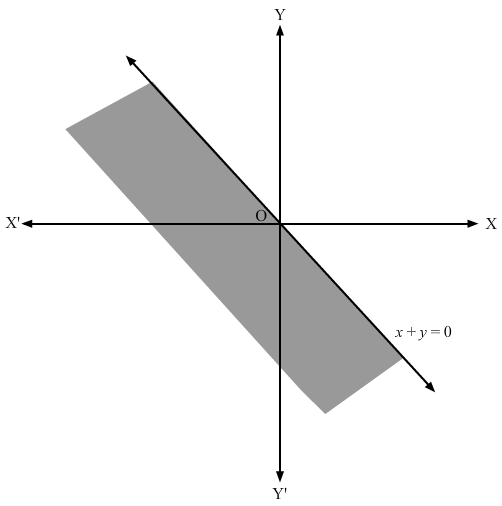Question 2 : Represent to solution set of each of the following in equations graphically in two dimensional plane:

x + 2y ≥6

Converting the in equation to equation, we obtain x + 2y = 6, i.e x + 2y −- 6 = 0.
Putting y = 0 and x = 0 in this equation, we obtain x = 6 and y = 3.
So, this line meets x-axis at (6,0) and y-axis at (0,3).
We plot these points and join them by a thick line. This divides the xy plane into two parts.
To determine the region represented by the given inequality, consider point O(0,0). Clearly,
(0,0) does not satisfy inequality x+2y≥6x+2y≥6.
So, the region that does not contain the origin is represented by the given inequality.
Hence, the shaded region is the solution to the in equation.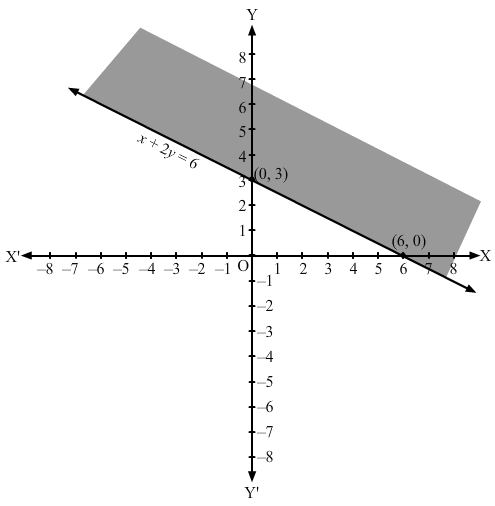Question 3 : Represent to solution set of each of the following inequations graphically in two dimensional
x + 2 ≥ 0

Converting the inequation to equation, we obtain x + 2 = 0, i.e x = −-2.
Clearly, it is a parallel line to y-axis at a distance of −-2 units from it. This line divides the xy plane into two parts, viz LHS  of x = −-2 and RHS of x = −-2. To determine the region represented by the given inequality, consider point O(0,0). Clearly, (0,0)  does not satisfy the inequality. So, the region that does not contain the origin is represented by the given inequality.
Hence, the shaded region is the solution to the inequation.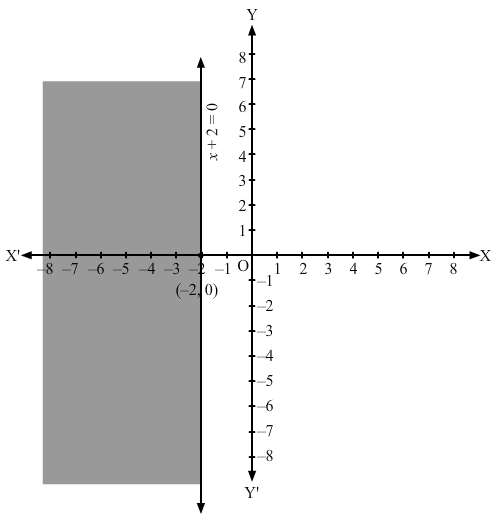Question 4 :
Represent to solution set of each of the following inequations graphically in two dimensional plane:
x − 2y < 0

Converting the inequation to equation, we obtain x −- 2y = 0
Putting y = 0 and x = 0 in this equation, we obtain x = 0 and y = 0 respectively. So, this line meets the x-axis at (0,0) and the y- axis at (0,0).
If x = 1, then y = 1/2.
So, we have another point (1,1/2).
We plot these points and join them by a thin line. This divides the xy plane into two parts.
We take a point (0, 2) and it does not satisfy the inequation.
Therefore, we shade the region which is opposite to the point (0, 2)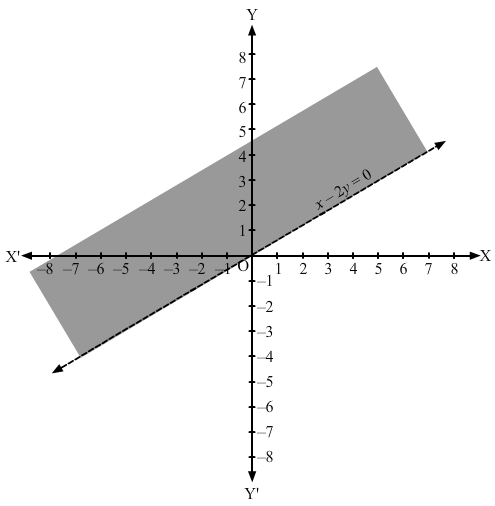Question 5 :
Represent to solution set of each of the following inequations graphically in two dimensional plane:
−3x + 2y ≤ 6

Converting the inequation to equation, we obtain −-3x + 2y = 6, i.e −-3x + 2y −- 6 = 0
Putting y = 0 and x = 0 in this equation, we obtain x = −-2 and y = 3 respectively. So, this line meets the x-axis at (−-2,0) and the y-axis at (0,3). We plot these points and join them by a thick line.This divides the xy plane in two parts. To determine the region represented by the given inequality, consider point O(0,0). Clearly, (0,0) satisfy the inequality −3x+2y≤6-3x+2y≤6. So, the region containing the origin is represented by the given inequality.
Hence, the shaded region is the solution to the inequation.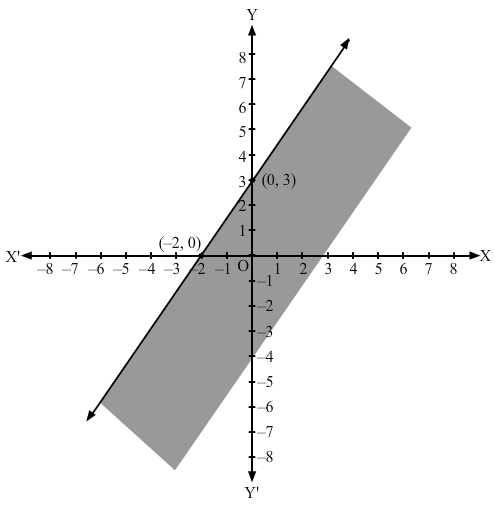Question 6 :
Represent to solution set of each of the following inequations graphically in two dimensional plane:
x ≤ 8 − 4y

Converting the inequation to equation, we obtain x + 4y −- 8 = 0
Putting y = 0 and x = 0 in this equation, we obtain x = 8 and y = 2 respectively. So, this line meets the x-axis at (8,0) and y-axis at (0,2). We plot these points and join them by a thick line. This divides the xy plane into two parts. To determine the region represented by the given inequality, consider point O(0,0). Clearly, (0,0) satisfies the inequality. So, the region containing the origin is represented by the given inequality.
Hence, the shaded region is the solution to the inequation.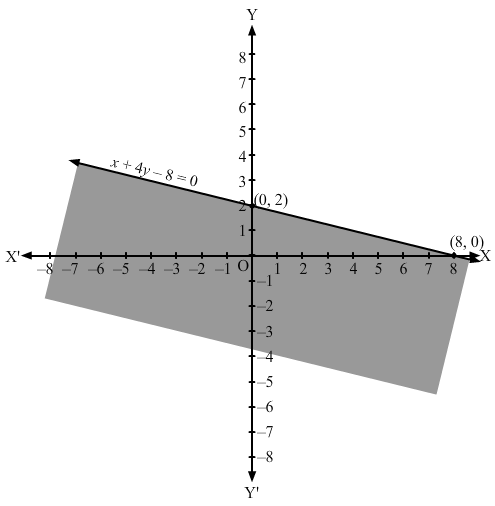Question 7 :
Represent to solution set of each of the following inequations graphically in two dimensional plane:
0 ≤ 2x − 5y + 10

Converting the inequation to equation, we obtain 2x − 5y+10 = 0
Putting y = 0 and x = 0 in this equation, we obtain x = −- 5 and y = 2 respectively.
So, this line meets the x-axis at (−-5,0) and the y-axis at (0,2).
We plot these points and join them by a thick line.
This divides the xy plane into two parts. To determine the region represented by the given inequality, consider point O(0,0).
Clearly, (0,0) satisfies the inequality.
So, the region containing the origin is represented by the given inequality.
Hence, the shaded region is the solution to the inequation.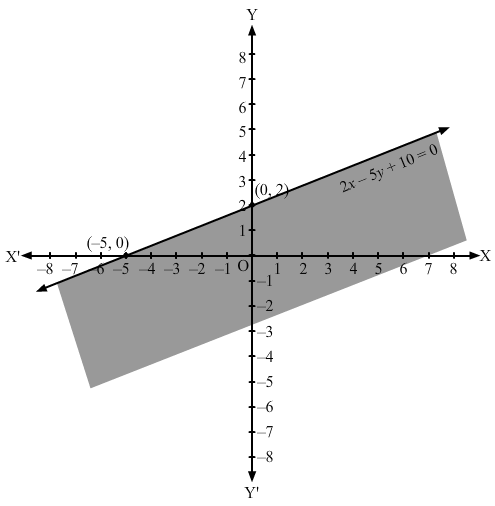Question 8 :
Represent to solution set of each of the following inequations graphically in two dimensional plane:
3y ≥ 6 − 2x

Converting the inequation to equation, we obtain 3y+2x −- 6 = 0
Putting y = 0 and x = 0 in this equation, we obtain x = 3 and y = 2 respectively.
So. this line meets the x-axis at (3,0) and y-axis at (0,2).
We plot these points and join them by a thick line.
This divides the xy plane into two parts. To determine the region represented by the given inequality, consider point O(0,0).
Clearly, (0,0) does not satisfy the inequality.
So, the region not containing the origin is represented by the given inequality.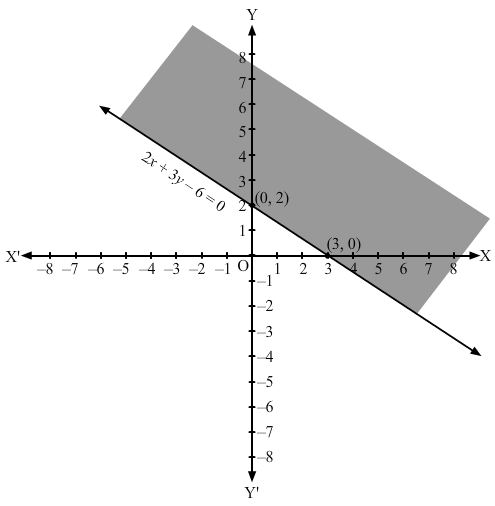Question 9 :
Represent to solution set of each of the following inequations graphically in two dimensional plane:
y ≥ 2x − 8

Converting the inequation to equation, we obtain 2x −- y −- 8 =0
Putting y = 0 and x = 0 in this equation, we obtain x = 4 and y = −- 8 respectively.
So, this line meets the x-axis at (4,0) and y-axis at (0, −-8).
We plot these points and join them by a thick line.This divides the xy plane into two parts.
To determine the region represented by the given inequality, consider point O(0,0).
Clearly, (0,0) does satisfy the inequality.
So, the region containing the origin is represented by the given inequality.
Hence, the shaded region is the solution to the inequation.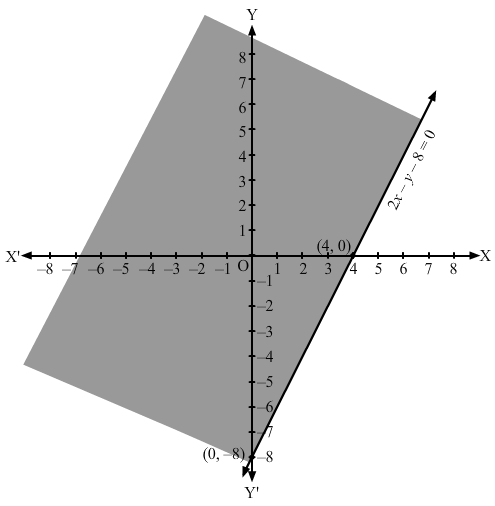Question 10 :
Represent to solution set of each of the following inequations graphically in two dimensional plane:
3x − 2y ≤ x + y − 8

Converting the inequation to equation, we obtain 3x −- 2y −- x −- y + 8 = 0, i.e 2x −- 3y + 8= 0
Putting  y =0 and x = 0 in this equation, we obtain x = −- 4 and y = 8/3 respectively.
So, this line meets the x-axis at (−-4, 0) and y-axis at (0,8/3).
We plot these points and join them by a thick line.
This divides the xy plane into two parts.
To determine the region represented by the given inequality, consider point O(0,0).
Clearly, (0,0) does not satisfy the inequality.
So, the region that does not contain the origin is represented by the given inequality.
Hence, the shaded region is the solution to the inequation.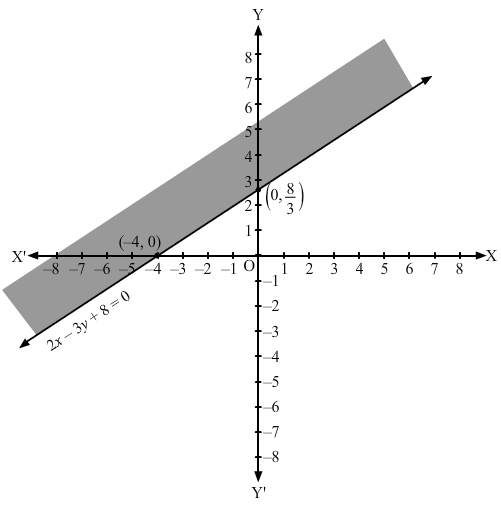Todays Deals### RD Chapter 15- Linear Inequations Ex-15.5 Contributorskrishan

Name:
Email:

# Latest News# 9000 interview questions in different categories